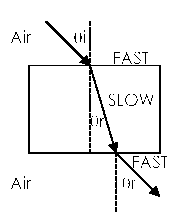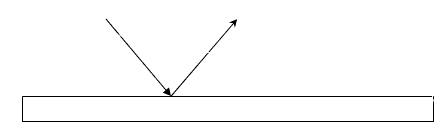## Wave Quiz

### Gapfill exercise

Enter your answers in the gaps. When you have entered all the answers, click on the "Check" button.

Wave Quiz 2 Tips

1. What is the angle of incidence (theta i) for the refraction shown below? degrees What is the angle of refraction of light IN the medium (theta r) ? degrees2. The tallest person that can see her whole body in a .4 meter mirror is meters Draw a picture

3. Light starts in medium x and enters water at an angle of 20 degrees. The angle of refraction is 40 degrees. What is the index of refraction of medium x ?   (3 significant figures)

4. What kind of reflection produces an image?

6. What kind of reflection doesn’t produce an image?

7. What is the angle of incidence for the reflection drawn below ? degrees and the angle of reflection? degrees .8. In refraction, if the angle of refraction is smaller than the angle of incidence, what does that tell you about the speed of the second medium? The second medium's speed is . the index of refraction of the second medium? The second medium's index of refraction is .

9.  What is the speed of light in Lucite? x 10^ 8 m/s

10. Which medium on your reference table is the slowest? fastest?
11.  What phenomena does one observe if light in a medium comes in at an angle greater than its critical?

total internal